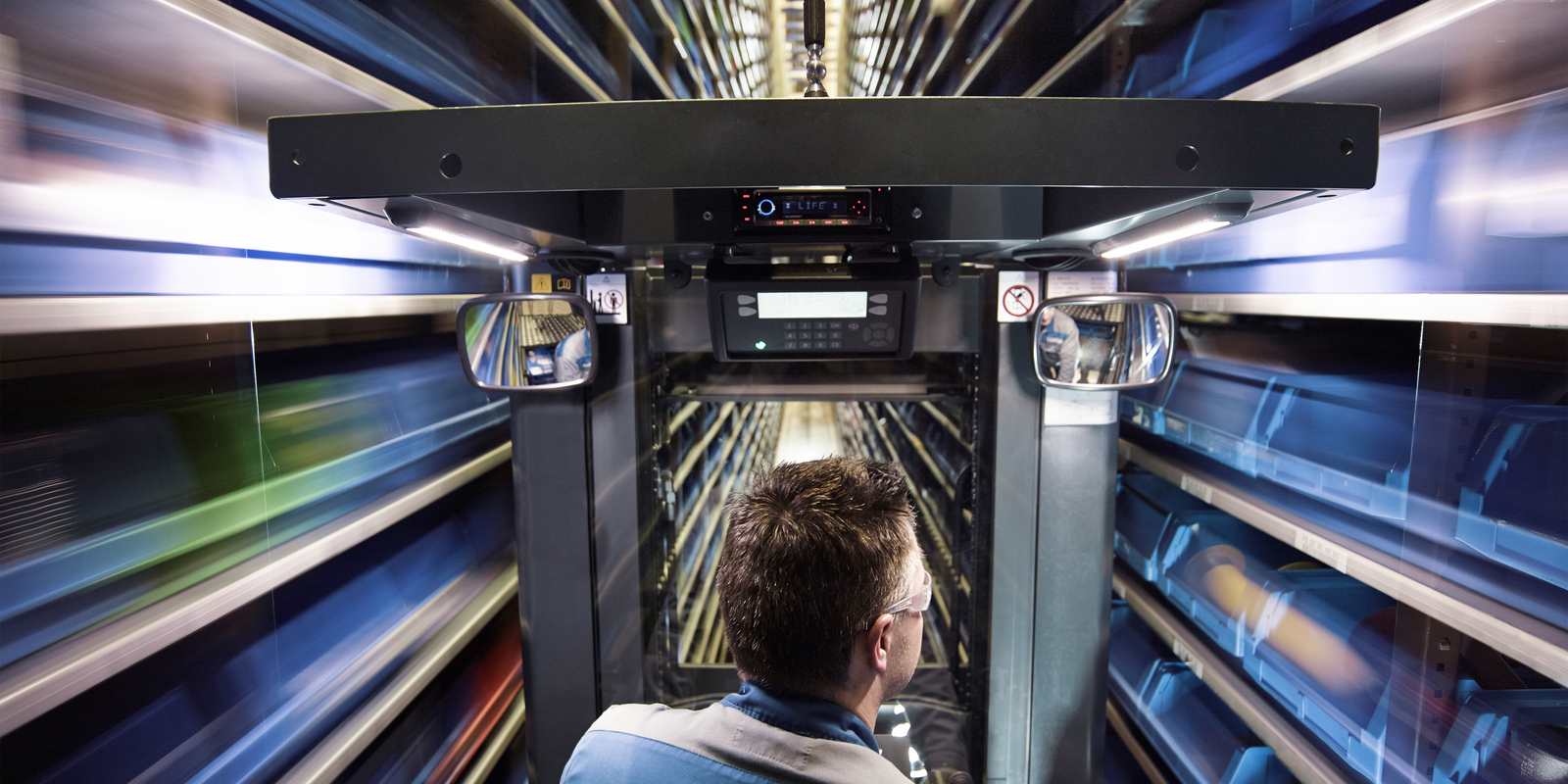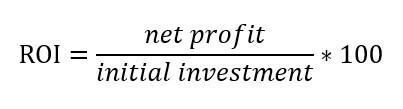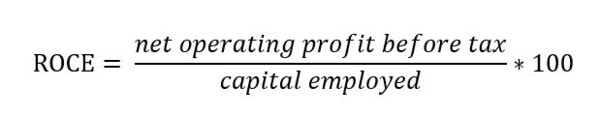# Financial metrics for evaluating warehouse projects: ROI and ROCEMay 2, 2023By Mike Weeks, Solution Specialist - Distribution Industries

2nd in Warehouse Management & Distribution series: The pros and cons of using return on investment versus return on capital employed as criteria

In the first post of this series, we talked briefly about the decision on when to implement a warehouse management system (WMS) or a warehouse control systems (WCS) with automation. In this post, we explore the potential financial metrics for evaluating warehouse projects and discuss the advantages and disadvantages.

Warehouse automation can help companies optimize their operations, reduce labour costs, and improve accuracy. However, before investing in any form of automation, it's essential to evaluate the financial viability of the investment.

## ROI (return on investment)

ROI measures the profitability of an investment relative to the initial investment cost. It is a commonly used financial metric and is easy to understand. The higher the ROI, the more profitable the investment is.

For a WMS or WCS investment, ROI may be more appropriate if the objective is to evaluate the financial viability of the investment, especially if the focus is on short-term profitability. ROI focuses on the project's profitability, regardless of how you finance the project.

To calculate ROI, you would divide the net profit of the investment by the initial investment cost and then multiply by 100 to get a percentage.Here's an example:Assume that a company is considering investing in a WMS that costs £500,000. The company estimates that the WMS will generate £750,000 in additional revenue and reduce labour costs by £200,000 per year. The company's net profit from the WMS investment would be £450,000 (£750,000 + £200,000 - £500,000). The ROI for the WMS investment would be 90% (£450,000 / £500,000 x 100%).

## ROCE (return on capital employed)

ROCE measures the efficiency of using all capital (debt and equity) to generate profit. It is a more complex metric than ROI as it includes all the capital employed to generate the profit. As a result, ROCE is useful when comparing the profitability of projects with different capital structures.

A WMS could be either an outright purchase or subscription-based software, or a WCS with automation investment might be financed with a loan. In this case, ROCE may be a more appropriate metric to evaluate the efficiency of the investment and to compare the profitability of the investment to other competing investments with different capital structures. In addition, ROCE considers the total capital employed in generating profits, which can help evaluate the efficiency of the investment.

To calculate ROCE:Here's an example:
Assume that a company is considering investing in a WCS that costs £1,000,000. The company estimates that the WCS will generate £1,500,000 in additional revenue and reduce labour costs by £300,000 per year. The company's net operating profit before tax from the WCS investment would be £800,000 (£1,500,000 + £300,000 - £1,000,000). The total capital employed in the project is £2,000,000 (£1,000,000 for the WCS and £1,000,000 for other capital investments). The ROCE for the WCS investment would be 40% (£800,000 / £2,000,000 x 100%).

Determining the numerator and denominator for ROCE

The challenge in calculating ROCE for a warehouse improvement project is determining the relevant figures for the numerator (net operating profit before tax) and the denominator (total capital employed).

Here are some potential metrics you could use for each:

Net operating profit before tax: This figure represents the profit generated by the warehouse operations before accounting for taxes and interest. To calculate this figure, you could take the revenue generated by the warehouse operations and subtract the cost of goods sold and any operating expenses such as rent, labour, and maintenance costs. If you compare different warehouse improvement options, you could estimate the change in revenue and costs for each option and use those figures to calculate the net operating profit before tax of each.

Total capital employed: This figure represents the total amount of capital invested in the warehouse, including equity and any debt used. To calculate this figure, you would add the full value of all assets used in the warehouse (such as inventory, equipment, and property) and subtract any liabilities (such as outstanding loans or lease payments). If you are comparing different warehouse improvement options, you would need to consider the additional capital investments required for each option, such as the cost of implementing a WMS or the cost of automation technology.

Once you have determined the relevant figures for both the numerator and denominator, you can use the formula to calculate the ROCE and compare the results for each warehouse improvement option.

## In conclusion

Evaluating the financial viability of warehouse automation investments is essential before deciding to implement a WMS or a WCS. ROI and ROCE are two financial metrics that companies can use to evaluate the profitability and efficiency of their investments. ROI measures the profitability of the investment relative to the initial investment cost, while ROCE measures the efficiency of using all capital to generate profit. Both metrics provide useful information and can be used in combination to gain a more comprehensive understanding of the investment.

When calculating ROCE, determining the relevant figures to use for both the numerator (net operating profit before tax) and the denominator (total capital employed) can be a challenge. Companies should consider the metrics and estimate the change in revenue and costs for each option when comparing different warehouse improvement options.

Read the 1st post: WMS vs WCS: Which one is right for your warehouse operations?

Filed Under
Industries
Products
Region